# ISEE Middle Level Math : How to find the part from the whole with percentage

## Example Questions

← Previous 1 3 4 5 6 7 8 9

### Example Question #1 : How To Find The Part From The Whole With Percentageis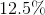of what number?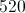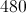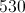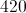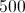Explanation:

Ifisof a number,, then we can set up an equation. First, we convertto a decimal,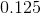. Then, we can formulate the equation below.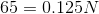Solve forby dividing both sides of the equation by.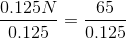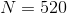### Example Question #2 : How To Find The Part From The Whole With Percentage

Which is larger?

a. 10% of 90

b. 5% of 160

c. 20% of 50

d. 30% of 40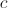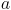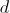Explanation:

First find the percentage amount for each choice by multiplying the number by the percentage amount

a.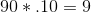b.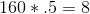c.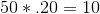d.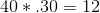Then compare the amounts to find the largest.

A laptop computer is on sale for 40% less than the original price. If the original price is $345.00, what is the laptop's current sale price? Possible Answers: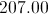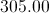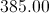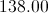Correct answer:Explanation: First, multiply the original price by the sale percentage: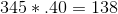Then, subtract that amount from the original price to get the sale price: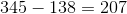. The laptop's current sale price is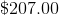### Example Question #1 : How To Work With Percentages Helen wants to buy a dress for a party. It is on sale for 37% off. How much does the dress currently costs, if its original price was$45.00?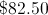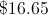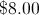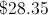Explanation:

First, multiply 45 by 0.37: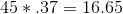Then, subtract that amount from 45.00 to find the sale price: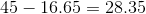Answer: The dress' sale price is $28.35. ### Example Question #5 : How To Find The Part From The Whole With Percentage A restaurant is having a sale and lowering their prices by 20%. If an average meal for two normally costs$62.00, what will it costs under the current sale?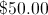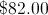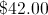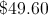Explanation:

Multiply: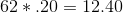Then, subtract that amount from the original price: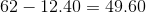Answer: $49.60 ### Example Question #6 : How To Find The Part From The Whole With Percentage Candy bought a sweater and a beanie on sale for 20% off. If the sweater cost$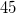and the beanie cost $, how much did Candy spend? Possible Answers:$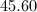$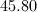$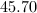$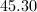$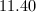$Explanation: In order to find how much money Candy spent, we must first figure out the cost of the merchandise before the discount. We do this by adding the prices together.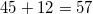Next we must find 20% of$57.00.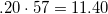Since 20% is the percentage Candy saved, we must subtract $11.40 from the original price.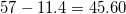So Candy spent$45.60.

### Example Question #7 : How To Find The Part From The Whole With Percentage

75 is 60% of what number?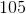Explanation:

Set up the proportion statement and solve forby cross-multiplying: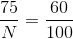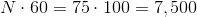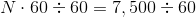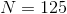### Example Question #8 : How To Find The Part From The Whole With Percentage

What is 120% of 120?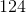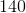Explanation:

Rewrite 120% as a decimal by writing 120 with a decimal point, then shifting it two spaces left: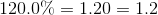Multiply this by 120: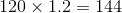### Example Question #9 : How To Find The Part From The Whole With Percentage

What is 225% of 225?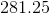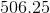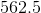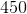Explanation:

Rewrite 225% as a decimal by writing 225 with a decimal point, then shifting it two spaces left: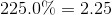Multiply this by 225: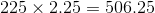### Example Question #10 : How To Find The Part From The Whole With Percentage

What is 77% of 77?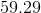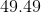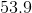Explanation:

Rewrite 77% as a decimal by writing 77 with a decimal point, then shifting it two spaces left: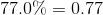Multiply this by 77: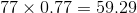← Previous 1 3 4 5 6 7 8 9